E58 Control Theory: Lab 2
System Identification for a DC motor
in partnership with aron dobos, david luong and mark piper
January 30, 2005

Abstract

In this lab the system parameters for a DC motor system were determined.  The motor was attached to a tachometer, flywheel, and a load motor.  Data was acquired for the unloaded spin up of the motor, as well as the response of the system upon the application of a load.  The data values compared favorably to those determined in previous years.

Derivations

To measure the constants km and R, we can use equations 3 and 5,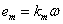(1)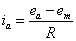(2)

Divide by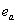,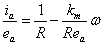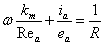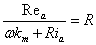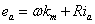Finally,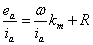Since we have measured values for,, and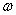, we can plot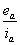versus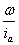to determine the slope and intercept, which reveals the constant values of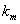and R.

To derive B, tf, and kw we use the equation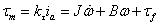For steady state= 0, so we have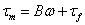If we plot the graph of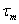versus, and plot a best fit line we get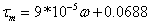This corresponds to a B value of  9*10-5. and a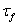value of 0.0688.

Once we know, we can find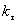using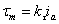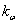is the slope of the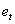vsgraph and is 0.0128.

Results

The system parameters for the DC motor are summarized in the graphs and tables below.  Setup 1 was used in determining the parameters.

Figure 1. Graph used to determine kw.Figure 2. Graph used to determine constants km and R.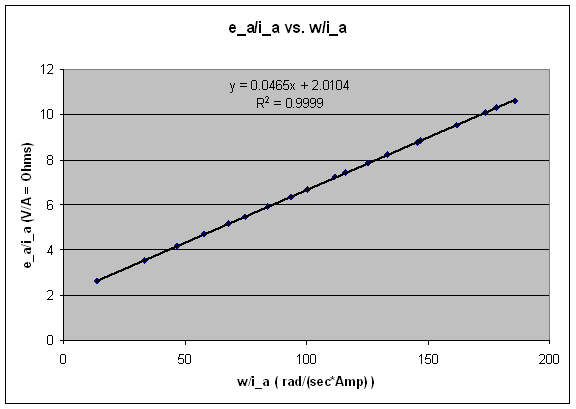Figure 3.  Graph used to determine B and Tf.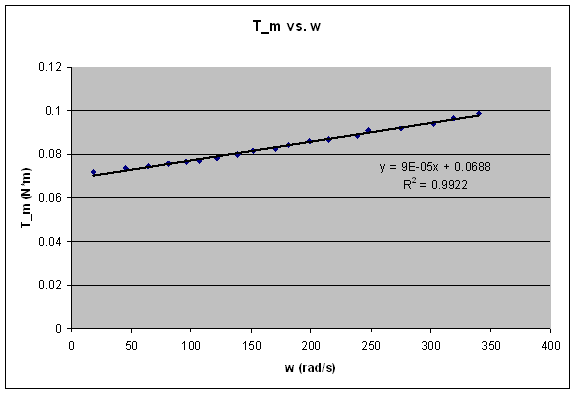Table 1. System Parameters

 kw B R km Tf kT 0.0128 9.00E-05 2.0104 0.0465 0.0688 0.054

Viscous Friction vs. Coulombic Friction

We estimate the speed at which the viscous friction is greater than the coulomb friction, that is, when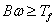.  The speed can be calculated by dividing Tf by B: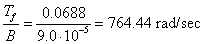The approximate tachometer voltage corresponding to this speed can be determined by using the best fit equation for the relationship between et  and w.  The best fit equation is: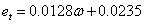.  Plugging in w calculated above, we get an expected tachometer voltage: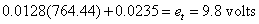This voltage is nearly double any tachometer voltage determined in the lab.  As such, we can assume that the Coulomb friction in the motor system dominates.

Calculating J from the Motor Spinup Data

Applying a step input of 18 volts to the motor, and acquiring data samples at 60 Hz, we obtained a curve for the tachometer voltage vs. time.  Given the form of the first order response of the system to an input, we fit the data with an appropriate exponential to determine the time constant.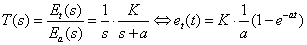where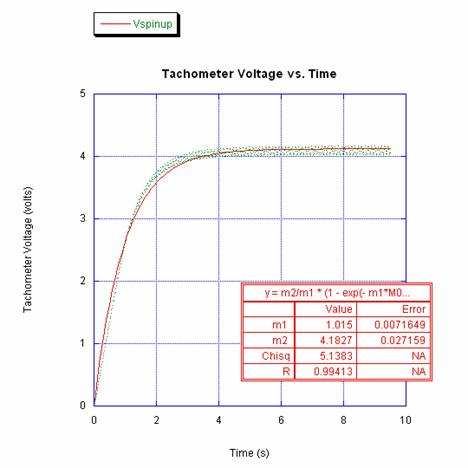Using the above equations and the best fit constants for the motor spin up obtained above, we calculate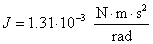System Transfer Function

The theoretical response of the system given the calculated parameters is shown below:Applying a step input of 2.5 volts, the result obtained in MATLAB is shown below, graphed next to the experimentally obtained data.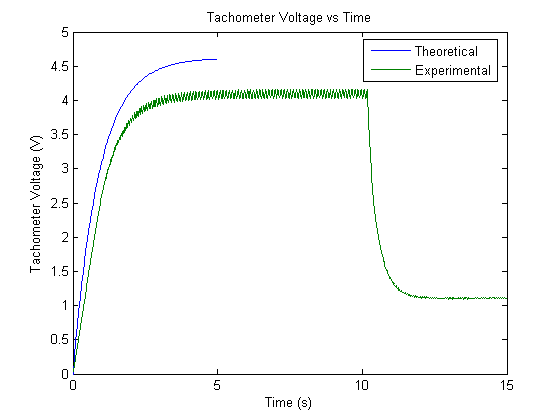The difference in steady state value can be accounted for by the nonlinear coulomb friction that the current transfer function neglects.

Throwing the switch to short out the leads of the load motor causes the rotation to slow down.  The tachometer voltage is shown below as a function of time.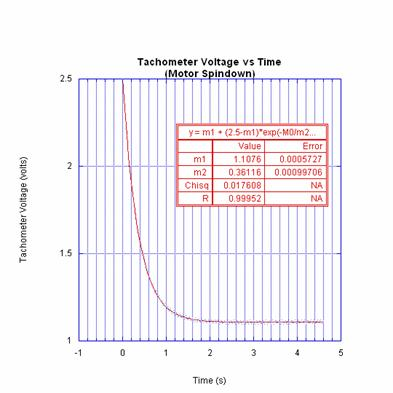For the motor spin down, we see that the final voltage is 1.1076 V, which is a 75.3 % drop from the original steady state voltage of 4.12 V.

Simulink Model Accounting for Coulomb Friction

The Simulink model including Coulomb friction is shown below.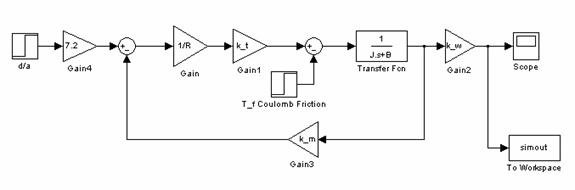Simulating the model and plotting it against the experimental data results in the graph below.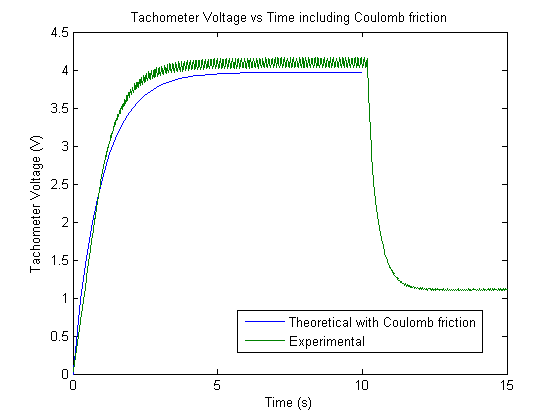Accounting for the Coulomb friction makes the theoretical data agree better with the experimental data.

Matlab Motor Control and Data Acquisition Control Code

`%%%%%%%%%%%%%%%%%%%%%%%%%%%%%%%%%%%%%%%%%%%%%%%%%%%%%%%%%%%%`
`% Engineering 58 Lab 2: System Identification of a DC Motor`
`% David Luong, Mark Piper, Kader, Aron Dobos`
`%%%%%%%%%%%%%%%%%%%%%%%%%%%%%%%%%%%%%%%%%%%%%%%%%%%%%%%%%%%%`
` `
`% Set up sampling parameters`
`clc;                    %Clear the display `
`SampleFreq=60;          %Sampling Frequency`
`NumPts=SampleFreq*15;   %Number of points in run (<=2000).`
`PreSample=0;            %Number of points before control`
`                        %  algorithm runs (this generates`
`                        %  baseline data);`
`InChan=0;               %Input channel to use on DAQ board`
`OutChan=0;              %Output channel to use on DAQ board`
`DT=1/SampleFreq;        %Sampling Interval`
` `
`%Setup the input channel`
`%You shouldn't need to change this.`
`Ain = analoginput('nidaq');`
`Ain.SampleRate=SampleFreq;`
`Ain.SamplesPerTrigger=NumPts;`
`Ain.InputType='SingleEnded';`
`Ain.BufferingConfig=[1  2000];`
`Ain.TransferMode='Interrupts';`
`addchannel(Ain,InChan);`
` `
`%Setup the output channel`
`%You shouldn't need to change this.`
`Aout = analogoutput('nidaq');`
`addchannel(Aout,OutChan);`
` `
`%%%%%%%%%%%%%%%%%%%%%%%%%%%%%%%%%%%%%%%%%%%%%%%%%%%%%%%%%%%`
`%%%%%%%% Predefine any arrays to make loop quicker %%%%%%%%`
`%%%%%%%%%%%%%%%%%%%%% Change as needed %%%%%%%%%%%%%%%%%%%%`
`%%%%%%%%%%%%%%%%%%%%%%%%%%%%%%%%%%%%%%%%%%%%%%%%%%%%%%%%%%%`
`OutData=zeros(NumPts,1);`
`%%%%%%%%%%%%%%%%%%%%%%%%%%%%%%%%%%%%%%%%%%%%%%%%%%%%%%%%%%%`
` `
`%Define Time Vector (time=0 after PreSample is done)`
`t=((1:NumPts)-PreSample)*DT;`
` `
`LastIndex=0;`
`start(Ain);`
` `
`% turn on the motor with a step input`
` `
`% apply a step input to the motor.  This applies 2.5*7.2 = 18 V`
`% to the motor leads.`
` `
`putsample(Aout, 2.5);`
`while (Ain.SamplesAcquired<NumPts),`
`    j=Ain.SamplesAcquired;`
` `
`    while(LastIndex==j),            %Wait for next datum.`
`        j=Ain.SamplesAcquired;`
`    end`
`    if ((j-LastIndex)~=1),          %Make sure no data was missed.`
`        disp(sprintf('Warning, datum skipped: %d, %d',LastIndex,j));`
`    end`
`    InData=peekdata(Ain,j);         %Retrieve most recent datum.`
` `
`    if (t(j)<0),        %If during PreSample, output 0.`
`        OutData(j)=0;`
`    else                %Else, do control algorithm`
`        OutData(j) = InData(j);`
`    end`
`    %%%%%%%%%%%%%%%%%%%%%%%%%%%%%%%%%%%%%%%%%%%%%%%%%%%%%%%%%%%`
` `
`    LastIndex=j;`
`end`
` `
`% turn off the motor`
`putsample(Aout,0);`
` `
`%Free up any memory that we used.`
`delete(Ain)`
`clear Ain`
`delete(Aout)`
`clear Aout`
` `
`plot(t,InData,t,OutData)`
`xlabel('Time');`
`ylabel('Voltage');`
`title('Tachometer Voltage vs time');`
`%legend('Vin','Vout');`
`save 'MyData' t InData OutData`